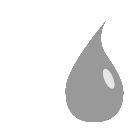# Blur nodeThis documentation is for version 4.0 of Blur (net.sf.cimg.CImgBlur).

## Description

Blur input stream or compute derivatives.

The blur filter can be a quasi-Gaussian, a Gaussian, a box, a triangle or a quadratic filter.

Note that the Gaussian filter  is implemented as an IIR (infinite impulse response) filter , whereas most compositing software implement the Gaussian as a FIR (finite impulse response) filter by cropping the Gaussian impulse response. Consequently, when blurring a white dot on black background, it produces very small values very far away from the dot. The quasi-Gaussian filter is also IIR.

A very common process in compositing to expand colors on the edge of a matte is to use the premult-blur-unpremult combination . The very small values produced by the IIR Gaussian filter produce undesirable artifacts after unpremult. For this process, the FIR quadratic filter (or the faster triangle or box filters) should be preferred over the IIR Gaussian filter.

References:

 I.T. Young, L.J. van Vliet, M. van Ginkel, Recursive Gabor filtering. IEEE Trans. Sig. Proc., vol. 50, pp. 2799-2805, 2002. (this is an improvement over Young-Van Vliet, Sig. Proc. 44, 1995)

 B. Triggs and M. Sdika. Boundary conditions for Young-van Vliet recursive filtering. IEEE Trans. Signal Processing, vol. 54, pp. 2365-2367, 2006.

 Nuke Expand Edges or how to get rid of outlines. http://franzbrandstaetter.com/?p=452

 Colour Smear for Nuke. http://richardfrazer.com/tools-tutorials/colour-smear-for-nuke/

Uses the ‘vanvliet’ and ‘deriche’ functions from the CImg library.

CImg is a free, open-source library distributed under the CeCILL-C (close to the GNU LGPL) or CeCILL (compatible with the GNU GPL) licenses. It can be used in commercial applications (see http://cimg.eu).

Input

Description

Optional

Source

No

Yes

## Controls

Parameter / script name

Type

Default

Function

Size / `size`

Double

x: 0 y: 0

Size (diameter) of the filter kernel, in pixel units (>=0). The standard deviation of the corresponding Gaussian is size/2.4. No blur is applied if size < 0.24 (Gaussian and quasi-Gaussian) or <= 1 (box, triangle and quadratic).

Uniform / `uniform`

Boolean

Off

Apply the same amount of blur on X and Y.

X derivation order / `orderX`

Integer

0

Derivation order in the X direction. (orderX=0,orderY=0) does smoothing, (orderX=1,orderY=0) computes the X component of the image gradient.

Y derivation order / `orderY`

Integer

0

Derivation order in the Y direction. (orderX=0,orderY=0) does smoothing, (orderX=0,orderY=1) computes the X component of the image gradient.

Border Conditions / `boundary`

Choice

Black

Specifies how pixel values are computed out of the image domain. This mostly affects values at the boundary of the image. If the image represents intensities, Nearest (Neumann) conditions should be used. If the image represents gradients or derivatives, Black (Dirichlet) boundary conditions should be used.
Black (black): Dirichlet boundary condition: pixel values out of the image domain are zero.
Nearest (nearest): Neumann boundary condition: pixel values out of the image domain are those of the closest pixel location in the image domain.

Filter / `filter`

Choice

Gaussian

Bluring filter. The quasi-Gaussian filter should be appropriate in most cases. The Gaussian filter is more isotropic (its impulse response has rotational symmetry), but slower.
Quasi-Gaussian (quasigaussian): Quasi-Gaussian filter (0-order recursive Deriche filter, faster) - IIR (infinite support / impulsional response).
Gaussian (gaussian): Gaussian filter (Van Vliet recursive Gaussian filter, more isotropic, slower) - IIR (infinite support / impulsional response).
Box (box): Box filter - FIR (finite support / impulsional response).
Triangle (triangle): Triangle/tent filter - FIR (finite support / impulsional response).

Expand RoD / `expandRoD`

Boolean

On

Expand the source region of definition by 1.5*size (3.6*sigma).

Crop To Format / `cropToFormat`

Boolean

On

If the source is inside the format and the effect extends it outside of the format, crop it to avoid unnecessary calculations. To avoid unwanted crops, only the borders that were inside of the format in the source clip will be cropped.

Alpha Threshold / `alphaThreshold`

Double

0

If this value is non-zero, any alpha value below this is set to zero. This is only useful for IIR filters (Gaussian and Quasi-Gaussian), which may produce alpha values very close to zero due to arithmetic precision. Remind that, in theory, a black image with a single white pixel should produce non-zero values everywhere, but a few VFX tricks rely on the fact that alpha should be zero far from the alpha edges (e.g. the premult-blur-unpremult trick to fill holes)). A threshold value of 0.003 is reasonable, and values between 0.001 and 0.01 are usually enough to remove these artifacts.

(Un)premult / `premult`

Boolean

Off

Divide the image by the alpha channel before processing, and re-multiply it afterwards. Use if the input images are premultiplied.

Invert Mask / `maskInvert`

Boolean

Off

When checked, the effect is fully applied where the mask is 0.

Mix / `mix`

Double

1

Mix factor between the original and the transformed image.